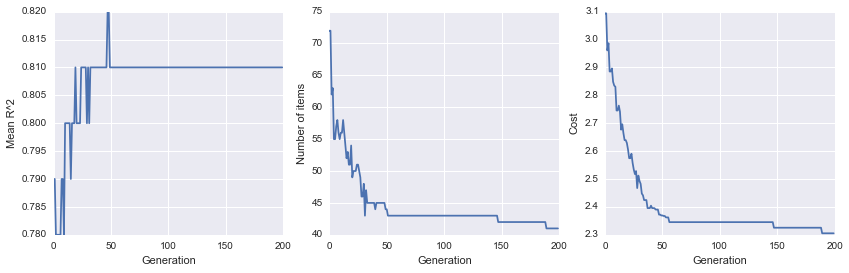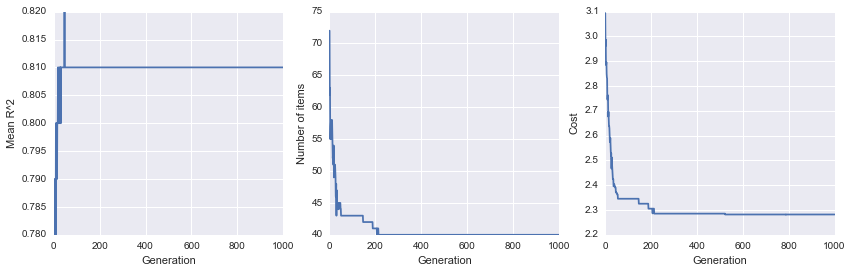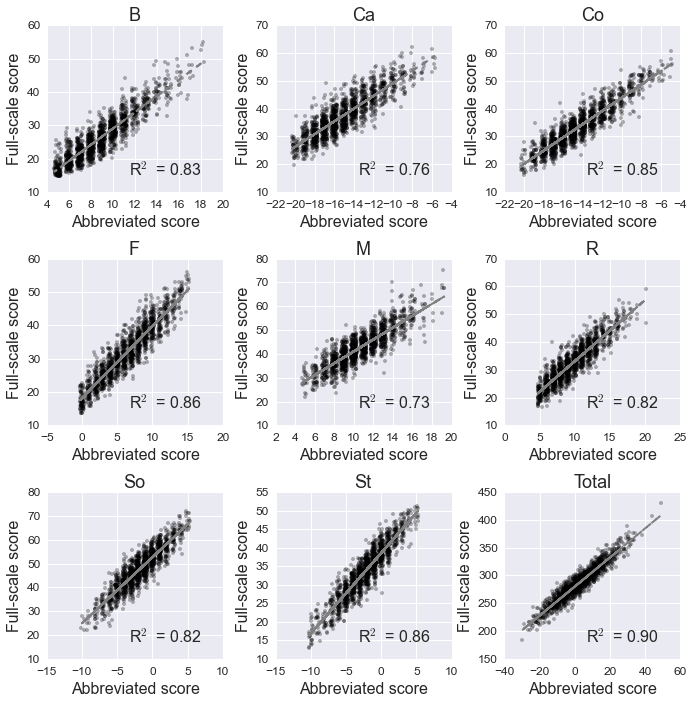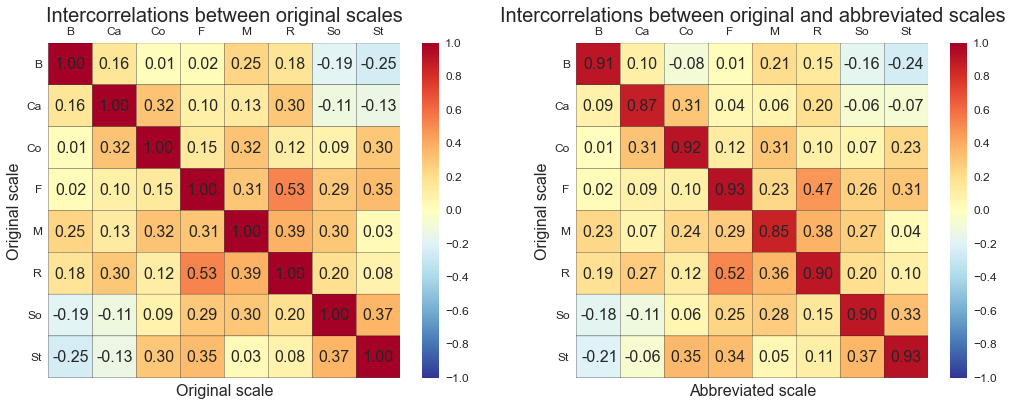# Abbreviating the PPI-R with precis¶

Tal Yarkoni (<a href=\"mailto:[email protected]\">Email</a> | <a href=\"http://talyarkoni.org\">Web</a> | <a href=\"http://twitter.com/talyarkoni\">Twitter</a> | <a href=\"http://github.com/tyarkoni\">GitHub</a>), June 2014

## What's precis?¶

Precis is a Python package for automated, genetic algorithm-based abbreviation of questionnaire measures. It's designed to solve a common problem many researchers in psychology and other fields face. Namely, that administering questionnaire measures is often an unpleasant process for both researchers and participants. It's unpleasant for researchers because questionnaire measures take up precious research time that could otherwise be used for other tasks. As for participants, well, spending a half hour or so filling out a few hundred likert items is not high up on most people's list of preferred activities. As a result, there's been an increasing push in recent years to develop abbreviated versions of common scales that do nearly as good a job measuring the construct of interest in considerably less time.

Precis is based on a recent Journal of Research in Personality paper that introduced a novel approach to the process of measure abbreviation. Instead of relying on conventional psychometric criteria iteratively evaluated by human investigators, the approach we proposed relies on a genetic algorithm to automatically "evolve" a good abbreviation. Our approach allows for considerable flexibility in the abbreviation process, as the user can define custom abbreviation and evaluation functions.

This notebook is more of an annotated example than a tutorial, and some basic knowledge of Python is assumed (though readers more familiar with R or Matlab will probably be okay too). We don't explain every step in detail, but the code below should provide a general sense of what precis does, and how you can use it to abbreviate other measures. All the files needed to run this example are contained within the examples folder of the precis github repository.

## The data¶

The data used in this example are from a forthcoming paper (Eisenbarth, Lilienfeld, & Yarkoni, 2014) in which we use precis to create a substantially shorter version of the Psychopathic Personality Inventory--Revised (PPI-R). The PPI-R, developed by Scott Lilienfeld and colleagues, is a widely used self-report measure of global psychopathy and its component traits. It consists of 154 items used to score a number of different psychopathy-related traits (Fearlessness, Stress immunity, Blame externalization, etc.). One practical limitation of the PPI-R is that it's a relatively long measure, precluding its use in situations where time is short. So in this notebook (and the paper the notebook is based on), we're going to generate a shorter version of the PPI-R and see how it performs.

The data in question come from three separate German-language samples. We've consolidated the data for all three samples in a single file located in the data folder. Subjects are in rows and PPI-R item scores are in columns. Scale scores aren't provided, as we'll generate them from the item scores using the scoring key located in the same directory.

## Preliminaries¶

First, let's import all of the precis modules and classes we'll need. We'll also import seaborn to give us nice plotting defaults.

In :
import seaborn as sns
from precis.base import Dataset, Measure, AbbreviatedMeasure
from precis.abbreviate import TopNAbbreviator
from precis.evaluate import YarkoniEvaluator
from precis.generate import Generator
from precis import plot as sp
%matplotlib inline


## Creating a Measure¶

The first thing we'll do is create a new Measure instance from PPI-R data. We'll pass in a text file containing the individual item scores. We'll also specify that all rows with any missing values (i.e., items that a participant didn't answer) should be dropped from analysis. We can also use mean imputation if we prefer, but since we have a pretty large sample in this case (> 1600 subjects), we can afford to be more conservative and simply eliminate subjects who didn't follow instructions 100% correctly.

Once the Measure instance is constructed, we'll then generate scale scores by passing a scoring key to the score() method. After that, we can display some useful summary information about the Measure by simply printing the object:

In :
# Initialize the Measure
ppi = Measure(X='data/PPI-R_German_data.txt', missing='drop')
# Generate scale scores from the PPI scoring key
ppi.score(key='data/PPI-R_scoring_key.txt', columns=['B','Ca','Co','F','M','R','So','St'], rescale=True)
# Display some information
print(ppi)

Number of items: 154
Number of scales: 8
Number of subjects: 1590

Scoring key:
B (15 items, R^2=1.00, alpha=0.87):	16, 18, 19, 38R, 40, 60, 62, 82R, 84, 90, 100R, 112, 122, 134, 144
Ca (19 items, R^2=1.00, alpha=0.81):	7, 29, 44R, 51R, 66, 73R, 88R, 89R, 99R, 101R, 108R, 111, 121R, 123R, 130R, 133R, 143R, 145R, 152R
Co (16 items, R^2=1.00, alpha=0.85):	5R, 9R, 27R, 31R, 49, 53R, 71R, 75R, 97R, 98R, 109R, 110R, 120R, 131, 142R, 153R
F (14 items, R^2=1.00, alpha=0.86):	3R, 12, 13, 25, 35, 47R, 57, 69R, 79R, 93, 115, 126, 137, 148
M (20 items, R^2=1.00, alpha=0.79):	1, 11, 17R, 23, 33, 39, 45, 55, 61, 67, 77, 83R, 92, 103, 114, 125, 132, 136, 147, 154
R (16 items, R^2=1.00, alpha=0.80):	4, 14, 15, 26, 36, 48, 58, 70, 80, 94, 104, 105, 116, 127, 138, 149
So (18 items, R^2=1.00, alpha=0.87):	2, 21R, 22R, 24R, 34, 41, 43, 46, 56, 63, 65R, 68R, 78, 85, 87R, 91, 113R, 135R
St (13 items, R^2=1.00, alpha=0.85):	6R, 10R, 28R, 32, 50R, 54, 72R, 76R, 96, 118, 119R, 140, 141R


As noted above, we're using a combined dataset made up of 3 separate German-language samples, for a total of n = 1,590 subjects. The scoring key displays the number and identity of items used to score each of 8 subscales on the PPI-R. R2 and Cronbach's alpha values are also displayed. (The R2 values are meaningless in this case. Normally, they would represent the convergent correlation between an abbreviated measure and the corresponding original measure, but in this case we're looking at the correlation between the original measure and itself, so all values are exactly 1.0.)

## Abbreviating the PPI-R¶

As you can see above, the canonical PPI-R has 154 items. That's a lot of items! It doesn't really need that many, does it? Well, let's find out! Below, we'll use precis to automatically abbreviate the PPI-R in just a few lines of code.

First, we'll initialize Abbreviator and Evaluator objects. As their name implies, these objects are charged, respectively, with abbreviating a measure, and evaluating the performance of that abbreviation. An explanation of what the constructor arguments mean is outside the scope of this example, but you can either take a look at the docstrings in abbreviate.py and evaluate.py, or read Yarkoni (2010). Actually, you don't have to explicitly initialize these objects, as sensible defaults will be selected for you if you don't. But it's still good practice, just so you're aware of what's going on.

In :
# Initialize an Abbreviator object
abb = TopNAbbreviator(max_items=5, min_r=0.2)
# Initialize an Evaluator object
ev = YarkoniEvaluator(item_cost=0.02)


Next, we'll initialize a new Generator instance. The Generator is the thing that does most of the dirty work for us. As its name suggests, it's responsible for generating a new abbreviated measure.

The Generator constructor takes a large number of optional arguments. You can specify which abbreviator and evaluator to use, and, if you're so inclined, pass a whole bunch of additional keywords in that tune the genetic algorithm. The latter parameters are passed directly to the DEAP package, which is what handles the actual evolutionary process. So if you want to tinker with the crossover and mutation rates, population size, etc., you can easily do that.

In :
gen = Generator(abbreviator=abb, evaluator=ev)


Once we initialize the Generator, creating an abbreviated measure is as easy as passing the original, full-length measure to the run() method, along with a specification of the number of generations to evolve a solution over. In this particular case, we'll also fix the random seed in order to ensure that we always get the same result when we re-run the code. If you don't set the seed, you'll tend to get somewhat different (though comparably good) abbreviations each time you run it.

Let's run the genetic algorithm for 200 generations:

In [ ]:
gen.run(ppi, n_gens=200, seed=64, resume=False)


This should take under 5 minutes on a reasonably new machine.

While our Generator evolves a shorter version of the PPI-R, it's also keeping track of some key statistics. When the abbreviation process is over, we might want to know how it actually did. For that, we have a handy plot_history() method. Right now the plot it produces is pretty ugly, but that's okay; the point here is more just to give you a sense of what's going on under the hood.

In :
_ = gen.plot_history(size=(14,4))The above figure simply summarizes three key performance metrics: (a) the mean R2 accounted for in the full-length scales by the abbreviated scales; (b) the number of items retained in the abbreviated measures; and (c) the total cost, or loss, of the best abbreviated measure in each generation. Note that the loss function depicted in the rightmost panel is monotonically decreasing (well, other than an occasional small increase due to the vagaries of random selection and recombination), whereas the other two metrics behave unpredictably, and depend largely on the parameters of the Abbreviator and the current DEAP settings. In this particular case, we can see that the number of retained items dropped steadily throughout the abbreviation run, whereas the mean R2 increased initially before hitting a plateau.

Interestingly, we can see that the total cost of the best measure in each generation is still decreasing even after 200 generations, which suggests that we should probably let the GA run for a longer period of time. Fortunately, the Generator is capable of running incrementally, so if we called run() again--passing resume=True this time--we can pick up where we left off in the evolutionary process, instead of having to start all over again. If we give it another 800 generations and then inspect the plot again, we can confirm that the loss appears to bottom out somewhere around generation 500, which means we're probably not going to see any further improvement in this particular run.

In :
gen.run(ppi, n_gens=800, seed=64, resume=True)
_ = gen.plot_history(size=(14,4))## Abbreviation results¶

Okay, now let's forget about the Generator object and take a look at what it returns once it's done running. We can assign a new variable, abb_ppi, which will contain the resulting abbreviated measure--an instance of class AbbreviatedMeasure. An AbbreviatedMeasure behaves just like a normal Measure for the most part, but also stores an internal reference to the Measure it originated from. Just as before, if we want to obtain some summary information about the new measure, we can simply print it:

In :
abb_ppi = gen.abbreviate()
print(abb_ppi)

Number of items: 40
Number of scales: 8
Number of subjects: 1590

Scoring key:
B (5 items, R^2=0.83, alpha=0.70):	18, 19, 40, 84, 122
Ca (5 items, R^2=0.76, alpha=0.68):	89R, 108R, 121R, 130R, 145R
Co (5 items, R^2=0.85, alpha=0.72):	27R, 75R, 97R, 109R, 153R
F (5 items, R^2=0.86, alpha=0.74):	12, 47R, 115, 137, 148
M (5 items, R^2=0.73, alpha=0.55):	33, 67, 77, 136, 154
R (5 items, R^2=0.82, alpha=0.68):	4, 36, 58, 80, 149
So (5 items, R^2=0.82, alpha=0.70):	22R, 34, 46, 87R, 113R
St (5 items, R^2=0.86, alpha=0.70):	10R, 32, 76R, 119R, 140

Original measure items kept: 4, 10, 12, 18, 19, 22, 27, 32, 33, 34, 36, 40, 46, 47, 58, 67, 75, 76, 77, 80, 84, 87, 89, 97, 108, 109, 113, 115, 119, 121, 122, 130, 136, 137, 140, 145, 148, 149, 153, 154


There are a couple of things to note here. First, our abbreviated measure is much shorter! We've gone from 154 items to 40 items in the space of just a few minutes. Second, that drop in length was achieved at the cost of relatively little loss of fidelity. We can see that the R2 values (reflecting the amount of variance in the original scale accounted for by the abbreviated scale) are universally quite high. The alphas, on the other hand, are arguably quite low by conventional psychometric standards. But for reasons I won't get into here, low internal consistency is actually a good thing in this context (see Yarkoni (2010) for discussion of this issue).

Before we go any further, let's save the result of our abbreviation for posterity. By default, calling save() on a Measure instance will save both a summary file containing the same information printed above, and a scoring key file that allows us to easily apply our abbreviated measure to new data:

In [ ]:
abb_ppi.save(path='abbreviations/', prefix='PPI', key=True, summary=True, pickle=False)


If we wanted to, we could also save a pickled version of the AbbreviatedMeasure instance itself, by passing pickle=True.

So far things seem to be working well. But appearances can be deceiving. It's not enough to know that the convergent correlation between the full-length and abbreviated PPI-R measures is high; we'd like to corroborate that conclusion with some additional analyses. Fortunately, precis has a number of plotting functions that can help give us a visual sense of how well we're doing.

First, we'll generate scatter plots displaying the relationship between the abbreviated and original scores for each PPI-R scale. The results--included in Eisenbarth, Lilienfeld, & Yarkoni (in press) as Figure 2--look pretty good:

In :
# Plot a set of scatterplots displaying correlation between abbreviated and original measure,
# one for each PPI-R scale.
sp.scale_scatter_plot(abb_ppi, rows=3, cols=3, trend=True, totals=True, jitter=0.3, alpha=0.3, size=(10,10))Another way to evaluate the fidelity of the abbreviated measure is to ask how well it preserves the relationship between the original scales. precis allows us to easily visualize this:

In :
sp.composite(gen, ['corr-original', 'corr-cross'], measure=abb_ppi, size=(16,6))Here we're taking advantage of a bit of magic in the composite() method, which is a high-level wrapper for a number of different plots. In this case, we're asking precis to generate two correlation matrices for us. The first one (left) displays the intercorrelation matrix for the 8 original PPI-R scales ('corr-original'); The second (right) displays the cross-correlations between the scales of the abbreviated and full-length PPI-R measures ('corr-cross'). If the abbreviation process was perfect, we would expect these two panels to look identical. In practice, they look nearly identical, which is about as much as we can realistically hope for.

The above figure is included as Figure 1 in Eisenbarth, Lilienfeld, & Yarkoni (in press).

That pretty much covers basic usage of precis. The rest of the code snippets below illustrate a few more advanced uses of precis.

### Generating multiple variants

First, suppose we want to create several different abbreviated measures, each with slightly different Abbreviator and Evaluator parameters. We can simply loop over the levels of our parameters (in this case, max_items and item_cost), saving the output each time:

In [ ]:
# Create a full set of abbreviations...
for mi in [3,5,7,9]:
for ic in [0.02, 0.04, 0.06, 0.08]:
abb = TopNAbbreviator(max_items=mi, min_r=0.2)
ev = YarkoniEvaluator(item_cost=ic)
gen = Generator(abbreviator=abb, evaluator=ev)
gen.run(ppi, n_gens=1000, seed=64, resume=False)
am = gen.abbreviate()
am.save(path='abbreviations/', prefix='PPI_mi=%d_ic=%f' % (mi, ic), key=True, summary=True)


Here's some rather ugly code for reading in the summaries of all of those different measures and consolidating them in one summary table (Table 1 in Eisenbarth, Lilienfeld, & Yarkoni, in press):

In :
# Format all abbreviated measures into a summary table
import re
import pandas as pd
def format_table(files):
header = '\t'.join(['MI', 'IC', 'Items', 'Mean_R2', 'mean_alpha'])
for f in files:
m = re.search('mi\=(\d+)_ic\=([0-9\.]+)', f)
if not m: continue
mi, ic = m.groups()
n_items = int(re.search('Number of items:\s(\d+)', c).group(1))
scales = re.findall('^(.*)\s+\((\d+)\s.*R\^2\=([\d\.]+).*alpha\=([\d\.]+)', c, re.MULTILINE)
df = pd.DataFrame(scales, columns=['name', 'no_items', 'R^2', 'alpha']).convert_objects(convert_numeric=True)
vals = (int(mi), float(ic), n_items, df['R^2'].mean(), df['alpha'].mean())
line = '%d\t%.2f\t%d\t%.2f\t%.2f' % vals
table.append(line)
print('\n'.join(table))

from glob import glob
files = glob('abbreviations/*summary.txt')
format_table(files)

MI	IC	Items	Mean_R2	mean_alpha
3	0.02	24	0.72	0.61
3	0.04	24	0.71	0.61
3	0.06	23	0.70	0.60
3	0.08	22	0.68	0.57
5	0.02	40	0.81	0.69
5	0.04	39	0.81	0.68
5	0.06	34	0.78	0.66
5	0.08	31	0.75	0.64
7	0.02	54	0.86	0.72
7	0.04	48	0.84	0.72
7	0.06	44	0.82	0.69
7	0.08	31	0.72	0.63
9	0.02	67	0.90	0.76
9	0.04	55	0.86	0.75
9	0.06	42	0.77	0.71
9	0.08	22	0.65	0.56


### Testing performance in a new dataset

Here, we apply the 40-item PPI-R abbreviation we generated above to a new data set, in order to assess how well the abbreviation generalizes to a new population:

In :
# Load the new data into its own measure
eng_ppi = Measure(X='data/PPI-R_MTurk_data.txt', missing='drop')
# Generate full-length PPI-R scale scores using the original PPI-R scoring key
eng_ppi.score(key='data/PPI-R_scoring_key.txt', columns=['B','Ca','Co','F','M','R','So','St'], rescale=True)
# Get the 40-item scoring key we generated above and use it to abbreviate the new measure directly
ppi_40_key = abb_ppi.key
abb_eng = AbbreviatedMeasure(eng_ppi, select=abb_ppi.original_items, key=ppi_40_key)
# Print summary of abbreviated measure to evaluate performance relative to full-length measure
print(abb_eng)

Number of items: 40
Number of scales: 8
Number of subjects: 229

Scoring key:
B (5 items, R^2=0.86, alpha=0.77):	18, 19, 40, 84, 122
Ca (5 items, R^2=0.73, alpha=0.55):	89R, 108R, 121R, 130R, 145R
Co (5 items, R^2=0.81, alpha=0.73):	27R, 75R, 97R, 109R, 153R
F (5 items, R^2=0.86, alpha=0.76):	12, 47R, 115, 137, 148
M (5 items, R^2=0.77, alpha=0.58):	33, 67, 77, 136, 154
R (5 items, R^2=0.79, alpha=0.74):	4, 36, 58, 80, 149
So (5 items, R^2=0.85, alpha=0.82):	22R, 34, 46, 87R, 113R
St (5 items, R^2=0.92, alpha=0.84):	10R, 32, 76R, 119R, 140

Original measure items kept: 1, 2, 3, 4, 5, 6, 7, 8, 9, 10, 11, 12, 13, 14, 15, 16, 17, 18, 19, 20, 21, 22, 23, 24, 25, 26, 27, 28, 29, 30, 31, 32, 33, 34, 35, 36, 37, 38, 39, 40


Lastly, we can compute the convergent correlations and Cronbach's alphas for higher-order factors of the PPI-R, as well as total score. Because these are simple composites of the existing scales, we can just append them to the scoring key and rescore.

In :
import numpy as np

# Add derivative scales to the scoring key
fear_dom = np.sum(key[:,[3,6,7]], axis=1)
imp_anti = np.sum(key[:,[0,1,4,5]], axis=1)
total = np.sum(key, axis=1)
return np.hstack((key, fear_dom[:,None], imp_anti[:,None], total[:,None])).astype(int)

# Load full PPI and score
full_ppi = Measure(X='data/PPI-R_German_data.txt', missing='drop')
full_ppi.score(key=old_key, columns=['B','Ca','Co','F','M','R','So','St','FD','IA','Tot'], rescale=True)

# Abbreviate
with_factors = AbbreviatedMeasure(full_ppi, select=abb_ppi.original_items, key=new_key)
print with_factors

Number of items: 40
Number of scales: 11
Number of subjects: 1590

Scoring key:
B (5 items, R^2=0.83, alpha=0.70):	18, 19, 40, 84, 122
Ca (5 items, R^2=0.76, alpha=0.68):	89R, 108R, 121R, 130R, 145R
Co (5 items, R^2=0.85, alpha=0.72):	27R, 75R, 97R, 109R, 153R
F (5 items, R^2=0.86, alpha=0.74):	12, 47R, 115, 137, 148
M (5 items, R^2=0.73, alpha=0.55):	33, 67, 77, 136, 154
R (5 items, R^2=0.82, alpha=0.68):	4, 36, 58, 80, 149
So (5 items, R^2=0.82, alpha=0.70):	22R, 34, 46, 87R, 113R
St (5 items, R^2=0.86, alpha=0.70):	10R, 32, 76R, 119R, 140
FD (15 items, R^2=0.89, alpha=0.78):	10R, 12, 22R, 32, 34, 46, 47R, 76R, 87R, 113R, 115, 119R, 137, 140, 148
IA (20 items, R^2=0.85, alpha=0.71):	4, 18, 19, 33, 36, 40, 58, 67, 77, 80, 84, 89R, 108R, 121R, 122, 130R, 136, 145R, 149, 154
Tot (40 items, R^2=0.90, alpha=0.79):	4, 10R, 12, 18, 19, 22R, 27R, 32, 33, 34, 36, 40, 46, 47R, 58, 67, 75R, 76R, 77, 80, 84, 87R, 89R, 97R, 108R, 109R, 113R, 115, 119R, 121R, 122, 130R, 136, 137, 140, 145R, 148, 149, 153R, 154

Original measure items kept: 1, 2, 3, 4, 5, 6, 7, 8, 9, 10, 11, 12, 13, 14, 15, 16, 17, 18, 19, 20, 21, 22, 23, 24, 25, 26, 27, 28, 29, 30, 31, 32, 33, 34, 35, 36, 37, 38, 39, 40